Documentation

### This is machine translation

Mouseover text to see original. Click the button below to return to the English version of the page.

To view all translated materials including this page, select Country from the country navigator on the bottom of this page.

# locallapfilt

Fast local Laplacian filtering of images

## Syntax

``B = locallapfilt(I,sigma,alpha)``
``B = locallapfilt(I,sigma,alpha,beta)``
``B = locallapfilt(___,Name,Value)``

## Description

example

````B = locallapfilt(I,sigma,alpha)` filters the grayscale or RGB image `I` with an edge-aware, fast local Laplacian filter. `sigma` characterizes the amplitude of edges in `I`. `alpha` controls smoothing of details.```
````B = locallapfilt(I,sigma,alpha,beta)` filters the image using `beta` to control the dynamic range of `A`.```

example

````B = locallapfilt(___,Name,Value)` uses name-value pairs to control advanced aspects of the filter. Argument names can be abbreviated.```

## Examples

collapse all

Import an RGB image

`A = imread('peppers.png');`

Set parameters of the filter to increase details smaller than 0.4.

```sigma = 0.4; alpha = 0.5;```

Use fast local Laplacian filtering

`B = locallapfilt(A, sigma, alpha);`

Display the original and filtered images side-by-side.

`imshowpair(A, B, 'montage')`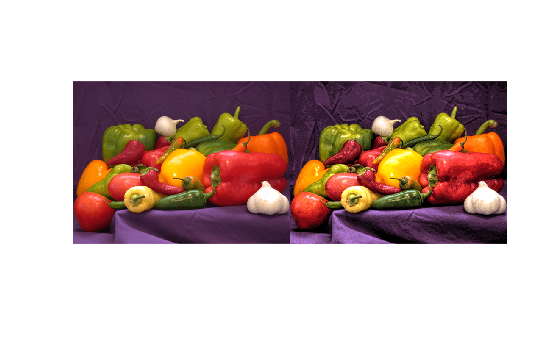Local Laplacian filtering is a computationally intensive algorithm. To speed up processing, `locallapfilt` approximates the algorithm by discretizing the intensity range into a number of samples defined by the '`NumIntensityLevels`' parameter. This parameter can be used to balance speed and quality.

Import an RGB image and display it.

```A = imread('peppers.png'); figure imshow(A) title('Original Image')```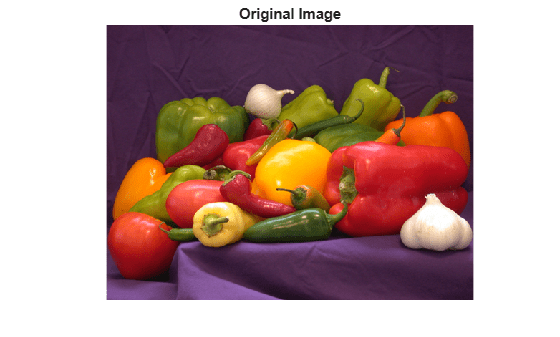Use a `sigma` value to process the details and an `alpha` value to increase the contrast, effectively enhancing the local contrast of the image.

```sigma = 0.2; alpha = 0.3;```

Using fewer samples increases the execution speed, but can produce visible artifacts, especially in areas of flat contrast. Time the function using only 20 intensity levels.

`t_speed = timeit(@() locallapfilt(A, sigma, alpha, 'NumIntensityLevels', 20)) `
```t_speed = 0.1374 ```

Now, process the image and display it.

```B_speed = locallapfilt(A, sigma, alpha, 'NumIntensityLevels', 20); figure imshow(B_speed) title(['Enhanced with 20 intensity levels in ' num2str(t_speed) ' sec'])```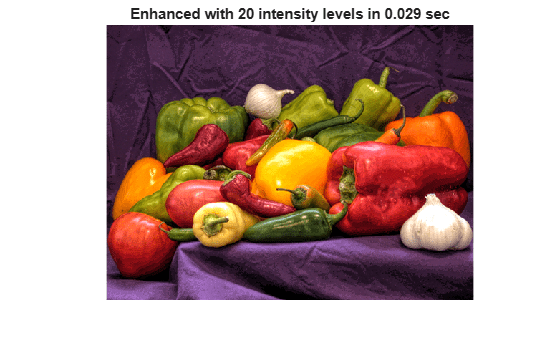A larger number of samples yields better looking results at the expense of more processing time. Time the function using 100 intensity levels.

`t_quality = timeit(@() locallapfilt(A, sigma, alpha, 'NumIntensityLevels', 100))`
```t_quality = 0.6107 ```

Process the image with 100 intensity levels and display it:

```B_quality = locallapfilt(A, sigma, alpha, 'NumIntensityLevels', 100); figure imshow(B_quality) title(['Enhancement with 100 intensity levels in ' num2str(t_quality) ' sec'])```Try varying the number of intensity levels on your own images. Try also flattening the contrast (with `alpha` > 1). You will see that the optimal number of intensity levels is different for every image and varies with `alpha`. By default, `locallapfilt` uses a heuristic to balance speed and quality, but it cannot predict the best value for every image.

Import a color image, reduce its size, and display it.

```A = imread('car2.jpg'); A = imresize(A, 0.25); figure imshow(A) title('Original Image')```Set the parameters of the filter to dramatically increase details smaller than 0.3 (out of a normalized range of 0 to 1).

```sigma = 0.3; alpha = 0.1;```

Let's compare the two different modes of color filtering. Process the image by filtering its intensity and by filtering each color channel separately:

```B_luminance = locallapfilt(A, sigma, alpha); B_separate = locallapfilt(A, sigma, alpha, 'ColorMode', 'separate');```

Display the filtered images.

```figure imshow(B_luminance) title('Enhanced by boosting the local luminance contrast')```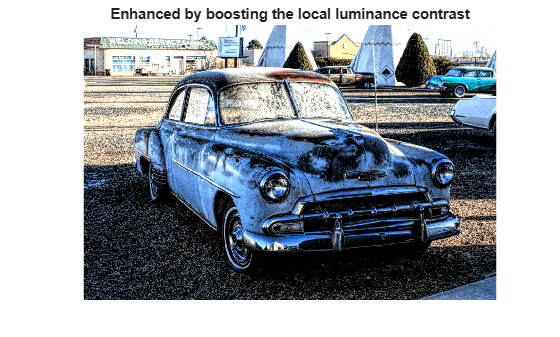```figure imshow(B_separate) title('Enhanced by boosting the local color contrast')```An equal amount of contrast enhancement has been applied to each image, but colors are more saturated when setting '`ColorMode`' to '`separate`'.

Import an image. Convert the image to floating point so that we can add artificial noise more easily.

```A = imread('pout.tif'); A = im2single(A);```

Add Gaussian noise with zero mean and 0.001 variance.

```A_noisy = imnoise(A, 'gaussian', 0, 0.001); psnr_noisy = psnr(A_noisy, A); fprintf('The peak signal-to-noise ratio of the noisy image is %0.4f\n', psnr_noisy); ```
```The peak signal-to-noise ratio of the noisy image is 30.0234 ```

Set the amplitude of the details to smooth, then set the amount of smoothing to apply.

```sigma = 0.1; alpha = 4.0;```

Apply the edge-aware filter.

```B = locallapfilt(A_noisy, sigma, alpha); psnr_denoised = psnr(B, A); fprintf('The peak signal-to-noise ratio of the denoised image is %0.4f\n', psnr_denoised);```
```The peak signal-to-noise ratio of the denoised image is 32.3065 ```

Note an improvement in the PSNR of the image.

Display all three images side by side. Observe that details are smoothed and sharp intensity variations along edges are unchanged.

```figure subplot(1,3,1), imshow(A), title('Original') subplot(1,3,2), imshow(A_noisy), title('Noisy') subplot(1,3,3), imshow(B), title('Denoised')```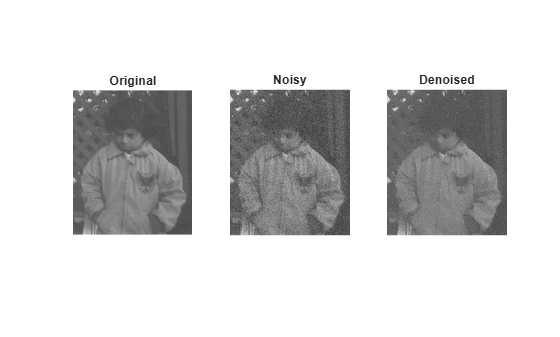Import the image, resize it and display it

```A = imread('car1.jpg'); A = imresize(A, 0.25); figure imshow(A) title('Original Image')```The car is dirty and covered in markings. Let's try to erase the dust and markings on the body. Set the amplitude of the details to smooth, and set a large amount of smoothing to apply.

```sigma = 0.2; alpha = 5.0;```

When smoothing (`alpha` > 1), the filter produces high quality results with a small number of intensity levels. Set a small number of intensity levels to process the image faster.

`numLevels = 16;`

Apply the filter.

`B = locallapfilt(A, sigma, alpha, 'NumIntensityLevels', numLevels);`

Display the "clean" car.

```figure imshow(B) title('After smoothing details')```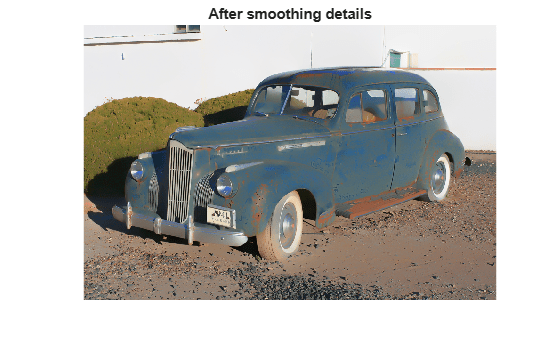## Input Arguments

collapse all

Image to filter, specified as a 2-D grayscale image of size m-by-n or a 2-D color image of size m-by-n-by-3.

Data Types: `single` | `int8` | `int16` | `uint8` | `uint16`

Amplitude of edges, specified as a non-negative scalar. `sigma` should be in the range [0, 1] for integer images and for single images defined over the range [0, 1]. For single images defined over a different range [`a`, `b`], `sigma` should also be in the range [`a`, `b`].

Data Types: `single` | `double` | `int8` | `int16` | `int32` | `int64` | `uint8` | `uint16` | `uint32` | `uint64`

Smoothing of details, specified as a positive scalar. Typical values of `alpha` are in the range [0.01, 10].

ValueDescription
`alpha` less than `1`Increases the details of the input image, effectively enhancing the local contrast of the image without affecting edges or introducing halos.
`alpha` greater than `1`Smooths details in the input image while preserving crisp edges
`alpha` equal to `1`The details of the input image are left unchanged.

Data Types: `single` | `double` | `int8` | `int16` | `int32` | `int64` | `uint8` | `uint16` | `uint32` | `uint64`

Dynamic range, specified as a non-negative scalar. Typical values of beta are in the range [0, 5]. `beta` affects the dynamic range of `A`.

ValueDescription
`beta` less than `1`Reduces the amplitude of edges in the image, effectively compressing the dynamic range without affecting details.
`beta` greater than `1`Expands the dynamic range of the image.
`beta` equal to `1`Dynamic range of the image is left unchanged. This is the default value.

Data Types: `single` | `double` | `int8` | `int16` | `int32` | `int64` | `uint8` | `uint16` | `uint32` | `uint64`

### Name-Value Pair Arguments

Specify optional comma-separated pairs of `Name,Value` arguments. `Name` is the argument name and `Value` is the corresponding value. `Name` must appear inside quotes. You can specify several name and value pair arguments in any order as `Name1,Value1,...,NameN,ValueN`.

Example:

Method used to filter RGB images, specified as one of the following values. This parameter has no effect on grayscale images.

ValueDescription
`'luminance'``locallapfilt` converts the input RGB image to grayscale before filtering and reintroduces color after filtering, which changes the contrast of the input image without affecting colors.
`'separate'``locallapfilt` filters each color channel independently.

Data Types: `char` | `string`

Number of intensity samples in the dynamic range of the input image, specified as `'auto'` or positive integer. A higher number of samples gives results closer to exact local Laplacian filtering. A lower number increases the execution speed. Typical values are in the range `[10, 100]`. If set to `'auto'`, `locallapfilt` chooses the number of intensity levels automatically to balance quality and speed based on other parameters of the filter.

Data Types: `single` | `double` | `int8` | `int16` | `int32` | `int64` | `uint8` | `uint16` | `uint32` | `uint64` | `char` | `string`

## Output Arguments

collapse all

Filtered image, returned as a numeric array the same size and class as the input image.

 Paris, Sylvain, Samuel W. Hasinoff, and Jan Kautz. Local Laplacian filters: edge-aware image processing with a Laplacian pyramid, ACM Trans. Graph. 30.4 (2011): 68.

 Aubry, Mathieu, et al. Fast local laplacian filters: Theory and applications. ACM Transactions on Graphics (TOG) 33.5 (2014): 167.